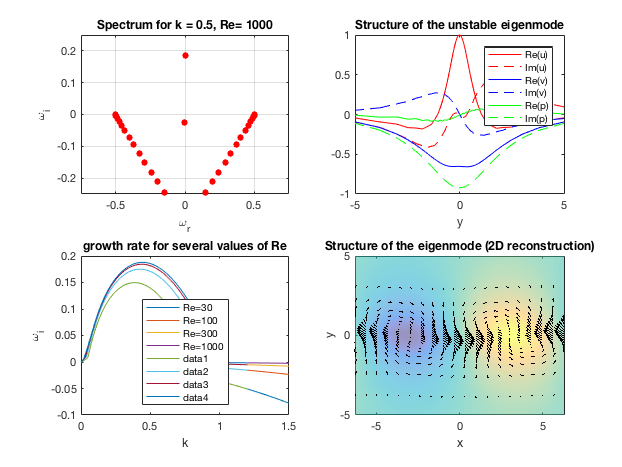# Kelvin-Helmholtz instability of a shear layer

(temporal analysis, viscous case, uvp formulation)

(This code is adapted from sandbox/easystab/kelvin_helmholtz_hermite.m from the easystab project).

We start from the linearised Navier-Stokes for a parallel base flow defined as U(y).

\displaystyle \begin{array}{l} \rho \partial_t u= -U \partial_y u - U_y v -\partial_x p +\mu\Delta u\\ \rho \partial_t v= -U \partial_y v - \partial_y p +\mu \Delta v\\ \partial_x u+ \partial_y v=0.\\ \end{array}

The base flow is \displaystyle U(y) = tanh(y)

We look for solutions under eigenmode form : \displaystyle [u,v,p] = [\hat u(y), \hat v(y), \hat p(y)] e^{i k x} e^{-\omega t}

We have seen that differentiation with respect to x ammounts to multiplication by i k, and differentiation with respect to t ammounts to multiplication by -i\omega, thus we have \displaystyle \begin{array}{l} -i \omega \hat{u}= -i k U \hat{u} - U_y \hat{v} - \hat{p}+\mu (-k^2 \hat{u}+\hat{u}_{yy})\\ - i \omega \hat{v}=-i k U \hat{u} -\hat{p}_y+\mu(-k^2 \hat{v}+\hat{v}_{yy}) \\ i k \hat{u}+\hat{v}_y=0\\ \end{array}

clear all; close all;
global y D DD w Z I U Uy discretization
% 'global' allows to use these objects in the function as well

alpha=0.5;    % the wave number
Re=1000;    % the Reynolds number
N=50;      % the number of grid points


### Derivation matrices

Here we use Chebyshev discretization with stretching. See differential_equation_infinitedomain.m to see how this works.

discretization = 'chebInfAlg'; %
[D,DD,w,y] = dif1D(discretization,0,1,N,0.9999);

Z=zeros(N,N); I=eye(N);
Ndim=N;

% base flow
U=tanh(y);
Uy=(1-tanh(y).^2);


We compute the eigenvalues/eigenmodes with the function KH, defined at the end of this program

[s,UU] = KH(alpha,Re,N);


### Plotting the spectrum

subplot(2,2,1);
plot(imag(s),real(s),'r.','markersize',20),hold on;
grid on
xlabel('\omega_r');
ylabel('\omega_i');
ylim([-.25 .25]);
xlim([-.75 .75]);
title('Spectrum for k = 0.5, Re= 1000');


### Plotting the eigenmode structure

% showing the velocity field
Nx=20;
indu=1:N; indv=indu+N; indp=indv+N;
q=UU(:,1);
q=q/q(N/2);%renormalisation so that u(0)=1
subplot(2,2,2);
plot(y,real(q(indu)),'r-',y,imag(q(indu)),'r--',y,real(q(indv)),'b-',y,imag(q(indv)),'b--',y,real(q(indp)),'g-',y,imag(q(indp)),'g--');
hold on;
legend('Re(u)','Im(u)','Re(v)','Im(v)','Re(p)','Im(p)');
xlabel('y');xlim([-5 5]);
title('Structure of the unstable eigenmode')

% expand to physical space
Lx=2*pi/alpha;  x=linspace(-Lx/2,Lx/2,Nx);
qphys=2*real(q*exp(i*alpha*x));

uu=qphys(indu,:);
vv=qphys(indv,:);
pp=qphys(indp,:);
% show the velocity field
sely=1:2:N;
subplot(2,2,4);
quiver(x,y(sely),uu(sely,:),vv(sely,:),0.2,'k'); hold on
axis([x(1),x(end),y(1),y(end)]);
xlabel('x'); ylabel('y'); title('Structure of the eigenmode (2D reconstruction)');
ylim([- 5 5]);

disp('Program paused ; type enter to launch construction of curves $\omega_i(k)$');
pause;


### Now we do loops over k and Re to plot the growth rate curves

\omega_i(k)

for Re = [30 100 300 1000]

alphatab = 0:.01:1.2;
lambdatab = [];
for alpha=alphatab
[s,UU] = KH(alpha,Re,N);
lambdatab = [lambdatab s(1)];
pause(0.1);
end
subplot(2,2,3);
plot(alphatab(1:length(lambdatab)),real(lambdatab));
hold on;
end

subplot(2,2,3);
legend('Re=30','Re=100','Re=300','Re=1000');
legend('Location','South');
xlabel('k');ylabel('\omega_i');
title('growth rate for several values of Re');

set(gcf,'paperpositionmode','auto');
print('-dpng','-r80','KH_temporal_viscous.png');** Figure : results for the Kelvin-Helmholtz instability (temporal) of a tanh shear layer

# Function KH

function [s,UU] = KH(alpha,Re,N)
global y D DD w Z I U Uy discretization % to use these objects within the function

% renaming the differentiation matrices
dy=D; dyy=DD;
dx=1i*alpha*I; dxx=-alpha^2*I;
Delta=dxx+dyy;


### System matrices

% the matrices
S=-diag(U)*dx+Delta/Re;
A=[ ...
S, -diag(Uy), -dx; ...
Z, S, -dy; ...
dx, dy, Z];
E=blkdiag(I,I,Z);

% Boundary conditions
if(strcmp(discretization,'her')==1)
indBC = [];
% No boundary conditions are required with Hermite interpolation which
% naturally assumes that the function tends to 0 far away.
else
% Dirichlet conditions are used for fd, cheb, etc...
III=eye(3*N);
indBC=[1,N,N+1,2*N];
C=III(indBC,:);
A(indBC,:)=C;
E(indBC,:)=0;
end

% computing eigenmodes

[UU,S]=eig(A,E);

% sort the eigenvalues by decreasing real part and remove the spurious ones
s=diag(S);  [t,o]=sort(-real(s)); s=s(o); UU=UU(:,o);
rem=abs(s)>1000; s(rem)=[]; UU(:,rem)=[];

end %function KH


# Exercices/Contributions

• Please compare the results with the inviscid ones obtained using the program KH_temporal_inviscid.m
• Please validate the results by comparing with the litterature (Drazin & Reid)
• Please try other discretisation methods, for instance Hermite —-> sandbox/easystab/kelvin_helmholtz_hermite.m
• Please look at the structure of the adjoint eigenmode and compute the nonnormality factor.

%}